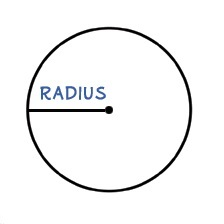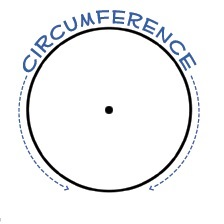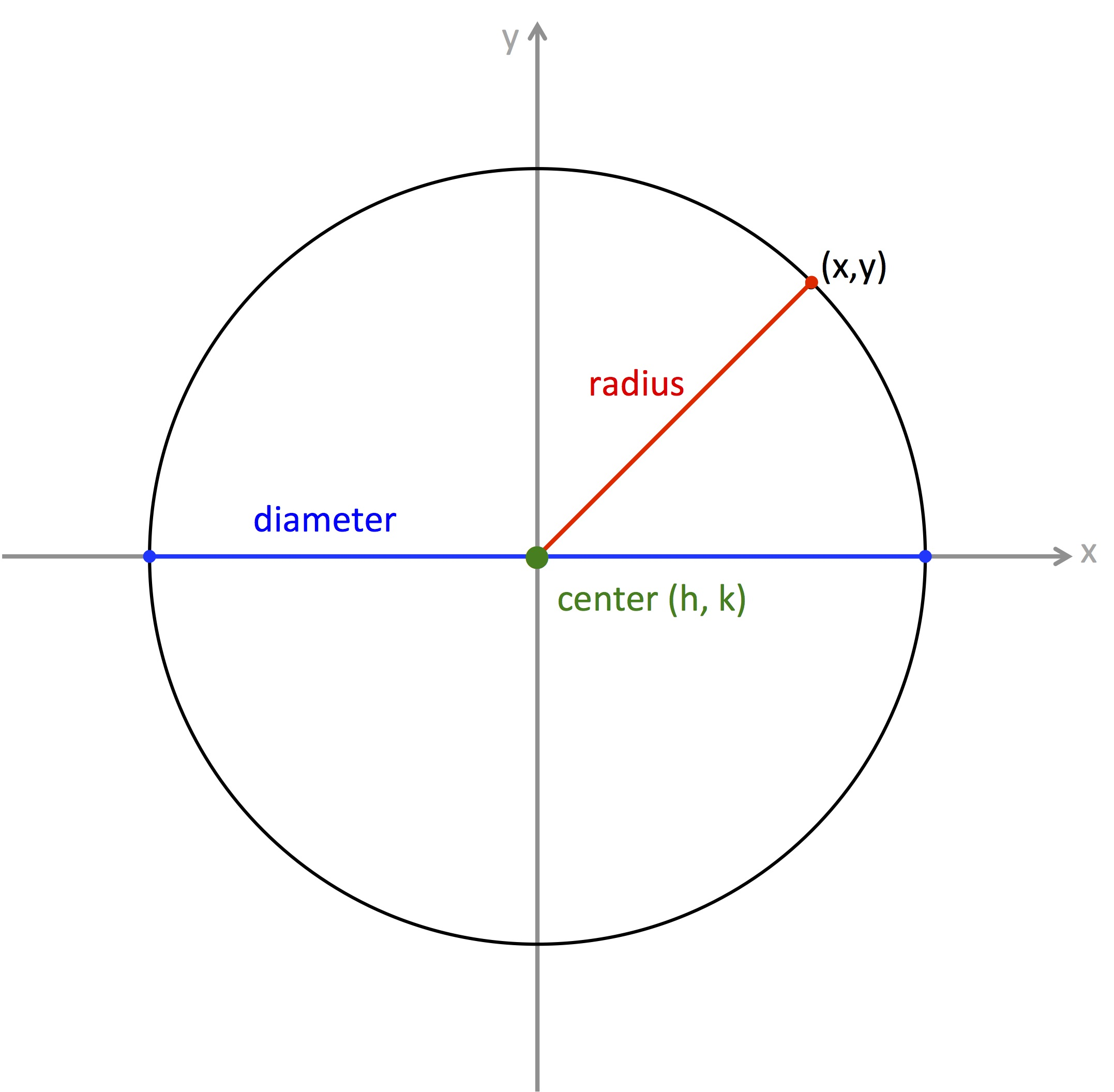# KS LearningGCSE Maths

### Notes on Number

KS Learning can provide extra lessons for maths from gcse maths tutors in London and help with gcse maths past papers, gcse maths revision notes, and gcse maths revision worksheets. Maths private tuition at its tuition centre can improve maths knowledge and performance through maths lessons, mathematics tutorials and maths tuition Twickenham.

### The Number Story (Once Upon a Sheep)

natural numbers - positive whole numbers: 1, 2, 3, 4, to infinity
counting numbers - zero and positive whole numbers: 0, 1, 2, 3, 4, to infinity
integers - positive and negative whole numbers plus zero : negative infinity ... -3, -2, -1, 0, 1, 2, 3, 4, to positive infinity
real numbers - all numbers on a number line including decimals, fractions, and surds

even numbers - divide by two exactly e.g. 2, 4, 6, 8
odd numbers - do not divide by two exactly e.g. 11, 13, 15, 17
prime numbers - have only two factors, one and themselves e.g. 2, 3, 5, 7, 11. Note that 1 is not a prime number because it has only one factor
surds - the root of a prime number or the product of different primes
fractions - a portion of a whole
square numbers - a number multiplied by itself produces a square number e.g. 4, 9, 16
cube numbers - a number multiplied by itself three times produces a cube number e.g. 8, 27, 64

factor - a number that divides exactly into another number e.g. 3 is a factor of 6
multiple - a number in the timetable of another number e.g. 12 is a multiple of 6
highest common factor - the largest number that divides into two or more numbers exactly e.g. 6 is the HCF of 24 and 30 (HCF)
lowest common multiple - the smallest number that two or more numbers divide into exactly e.g. 120 is the LCM of 24 and 30 (LCM)

vulgar fraction - expressed as a whole number over another whole number, respectively numerator and denominator, in which the denominator cannot be zero; also known as a simple or common fraction
proper fraction - a vulgar fraction where the numerator is smaller than the denominator
improper fraction - a vulgar fraction where the numerator is greater than the denominator; also called a top-heavy fraction

rational numbers - a rational number is any number that can be expressed as a fraction p/q where p and q are integers. Integers are rational numbers as they can be written over 1
irrational numbers - an irrational number cannot be written as a fraction. For example, the square root of 2 is an irrational number because it cannot be written as a ratio of two integers

### Mathematical Symbols

Maths makes use of the following symbols and terms

Symbol Meaning
= equal to
equivalent to
not equal to
< less than
> greater than
less than or equal to
greater than or equal to
approximately equal to
the sum of
α alpha
β beta
γ gamma
θ theta
infinity
therefore
therefore
Difference the result of substracting
Product the result of multiplying

### SI Prefixes

Prefixes are added to the base unit so 9Gm (giga metres) means 9 x 109 m = 9 000 000 000 m

Index Symbol Name
1012 T tera
109 G giga
106 M mega
103 k kilo
102 h hecto
101 da deca
base unit
10-1 d deci
10-2 c centi
10-3 m milli
10-6 µ micro
10-9 n nano
10-12 p pico

### Division Rules

Title Rule Examples
Divisible by 2 End with 0,2,4,6,8 254 and 4718 are divisible by 2
Divisible by 3 Sum of digits is divisible by 3 549 =5 + 4 + 9 = 18 = 1 + 8 = 9 is divisible by 3 hence 549 is divisible by 3
Divisible by 4 Last two digits divisible by 4 5648 here last 2 digits are 48 which is divisible by 4 hence 5648 is divisible by 4
Divisible by 5 Ends with 0 or 5 225 or 330 here last digit digit is 0 or 5 that mean both the numbers are divisible by 5
Divisible by 6 Divides by both 2 and 3 4536 here last digit is 6 so it divisible by 2 and 4+5+3+6=18=1+8=9 which is divisible by 3. Hence 4536 is divisible by 6
Divisible by 8 Last 3 digits divide by 8 746848 here last 3 digit 848 is divisible by 8 hence 746848 is also divisible by 8
Divisible by 10 End with 0 220,450,1450,8450 all numbers has a last digit zero it means all are divisible by 10

### Circle Terms

Term Explanation Illustration
semi-circle half a circleconcentric circles circles which share the same centrecircumference the perimeter of a circlearc a portion of the circumferenceminor arc an arc that is less than half the circumferencemajor arc an arc that is more than half the circumferenceradius the line from the centre of the cirle to the edge of the circlediameter the line from one side of the circle to the other through the centrechord a line from one side of the circle to the other side whose endpoints are on the edge of a circlesecant a line that pass through both sides of the circletangent a line that touches the circle at one point onlynormal a line at 90 degrees to the tangent at the point where it touches the circlesegment the portion of a circle between a chord and an arcsector the portion of a circle between two radii and an arcsubtended anglebisector a line that cuts another line in half### Circle Theorems

Number Theorem Diagram
Theorem 1 The angles on the cirumference of a circle are equal when subtended by the same arcTheorem 2 The angle at the centre of a circle, is twice the size of the angle at the circumference when subtended by the same arcTheorem 3 An angle on the circumference is 90 degrees when subtended by diameter of the circleTheorem 4 The opposite angles in a cycle quadrilateral always add up to 180 degrees.Theorem 5 A tangent to a circle is perpendicular to the radius at the point of contactTheorem 6 Tangents to a circle from the point they intersect to the points of contact are equal in lengthTheorem 7 The line joining the point where two tangents intersect to the centre of the circle, bisects the angle between the tangentsTheorem 8 If a radius bisects a chord, the angle between them will be at 90 degreesTheorem 9 The angle between a tangent and a chord through the point of contact is equal to the angle in the alternate segmentSites of Interest

### Confidence

A good tutor can build the confidence of a learner enabling subject success

### Skills

A private tutor can improve the skills a pupil needs to master a subject

### Progress

Regular tutoring can drive progress and better results in school subjects

### Support

Support can help students and parents make the right academic decisions